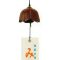The Japanese Bonsai specialist
Direct order Contact Help / Services NewsletterJapanese copper colour tulip wind bell g24

› Wind bells and chimesref. : 4656

11,00

Available quantity : 1Order

Description

5.4 * 4 cm. Tulip-shaped wind bell made in Japan. Place it near windows for a pleasant ring that creates a relaxing mood. Copper colour. The Tanzaku paper string, featuring Japanese script, blows gently in the wind. Made of durable Nambu cast iron.

#wind 6.4 #japanese 3.8 #colour 3.6 #copper 3.6 #tulip 3.5 #bell 3.4 #chimes 2.6 #bells 2.5 #made 2.4 #featuring 1.9

Sébastien GARBE 09-11-2021 23:28
Cloche discrète et très élégante

Formule
(( ROUND((CHAR_LENGTH(b.article_nom)-CHAR_LENGTH(REPLACE(b.article_nom, 'wind', '')))/LENGTH('wind')) + ROUND((CHAR_LENGTH(b.article_description)-CHAR_LENGTH(REPLACE(b.article_description, 'wind', '')))/LENGTH('wind')) ) * 4.4) + (( ROUND((CHAR_LENGTH(b.article_nom)-CHAR_LENGTH(REPLACE(b.article_nom, 'japanese', '')))/LENGTH('japanese')) + ROUND((CHAR_LENGTH(b.article_description)-CHAR_LENGTH(REPLACE(b.article_description, 'japanese', '')))/LENGTH('japanese')) ) * 3.8) + (( ROUND((CHAR_LENGTH(b.article_nom)-CHAR_LENGTH(REPLACE(b.article_nom, 'colour', '')))/LENGTH('colour')) + ROUND((CHAR_LENGTH(b.article_description)-CHAR_LENGTH(REPLACE(b.article_description, 'colour', '')))/LENGTH('colour')) ) * 3.6) + (( ROUND((CHAR_LENGTH(b.article_nom)-CHAR_LENGTH(REPLACE(b.article_nom, 'copper', '')))/LENGTH('copper')) + ROUND((CHAR_LENGTH(b.article_description)-CHAR_LENGTH(REPLACE(b.article_description, 'copper', '')))/LENGTH('copper')) ) * 3.6) + (( ROUND((CHAR_LENGTH(b.article_nom)-CHAR_LENGTH(REPLACE(b.article_nom, 'tulip', '')))/LENGTH('tulip')) + ROUND((CHAR_LENGTH(b.article_description)-CHAR_LENGTH(REPLACE(b.article_description, 'tulip', '')))/LENGTH('tulip')) ) * 3.5) + (( ROUND((CHAR_LENGTH(b.article_nom)-CHAR_LENGTH(REPLACE(b.article_nom, 'bell', '')))/LENGTH('bell')) + ROUND((CHAR_LENGTH(b.article_description)-CHAR_LENGTH(REPLACE(b.article_description, 'bell', '')))/LENGTH('bell')) ) * 3.4) + (( ROUND((CHAR_LENGTH(b.article_nom)-CHAR_LENGTH(REPLACE(b.article_nom, 'made', '')))/LENGTH('made')) + ROUND((CHAR_LENGTH(b.article_description)-CHAR_LENGTH(REPLACE(b.article_description, 'made', '')))/LENGTH('made')) ) * 2.4) + (( ROUND((CHAR_LENGTH(b.article_nom)-CHAR_LENGTH(REPLACE(b.article_nom, 'featuring', '')))/LENGTH('featuring')) + ROUND((CHAR_LENGTH(b.article_description)-CHAR_LENGTH(REPLACE(b.article_description, 'featuring', '')))/LENGTH('featuring')) ) * 1.9) + (( ROUND((CHAR_LENGTH(b.article_nom)-CHAR_LENGTH(REPLACE(b.article_nom, 'relaxing', '')))/LENGTH('relaxing')) + ROUND((CHAR_LENGTH(b.article_description)-CHAR_LENGTH(REPLACE(b.article_description, 'relaxing', '')))/LENGTH('relaxing')) ) * 1.8) + (( ROUND((CHAR_LENGTH(b.article_nom)-CHAR_LENGTH(REPLACE(b.article_nom, 'pleasant', '')))/LENGTH('pleasant')) + ROUND((CHAR_LENGTH(b.article_description)-CHAR_LENGTH(REPLACE(b.article_description, 'pleasant', '')))/LENGTH('pleasant')) ) * 1.8)

Secure paymentDelivery

Our logistic partners :04 74 55 23 48
Pépinière MAILLOT-BONSAÏ
Le Bois Frazy
01990 RELEVANT - FRANCE
on appointment